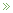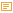• ## A PCA Puzzle

Posted by Konstantin 16.01.2012

This post presumes you are familiar with PCA.

Consider the following experiment. First we generate a random vector (signal) as a sequence of random 5-element repeats. That is, something like

(0.5, 0.5, 0.5, 0.5, 0.5,   0.9, 0.9, 0,9, 0.9, 0,9,   0.2, 0.2, 0.2, 0.2, 0.2,   ... etc ... )

In R we could generate it like that:

```num_steps = 50
step_length = 5;
initial_vector = c();
for (i in 1:num_steps) {
initial_vector = c(initial_vector, rep(runif(1), step_length));
}```

Here's a visual depiction of a possible resulting vector:

Next, we shall create a dataset, where each element will be a randomly shifted copy of this vector:

```library(magic) # Necessary for the shift() function
dataset = c()
for (i in 1:1000) {
shift_by = floor(runif(1)*num_steps*step_length) # Pick a random shift
new_instance = shift(initial_vector, shift_by)   # Generate a shifted instance
dataset = rbind(dataset, new_instance);          # Append to data
}```

Finally, let's apply Principal Component Analysis to this dataset:

`pca = prcomp(dataset)`

Question - how do the top principal components look like? Guess first, then read below for the correct answer.

Interestingly, the major principal components are all pretty much sine- and cosine waves

Moreover, the wave frequencies of the top-scoring principal components (4 and 7 cycles per signal in the example above) correspond exactly to the largest components of the Fourier transform of the initial signal. Observe:

```fourier = fft(initial_vector)
barplot(abs(fourier)[2:16])```

The observation bears some practical importance. Datasets that contain many "shifted copies" of otherwise similar signals are not impossible to come by. You will see those if you are studying sets of time series, which all mainly differ by the "starting time" of some event. Applying PCA to such a dataset will almost certainly result in the extraction of sinewaves, which is a variant of spectral decomposition.

Why does it work this way? I'll leave it for you to wonder. Hint - it has something to do with eigensignals of linear time-invariant systems.

Posted by Konstantin @ 3:05 am

Tags: , ,

Please note: Comment moderation is enabled and may delay your comment. There is no need to resubmit your comment.

### Calendar

January 2012
M T W T F S S
« Dec   May »
1
2345678
9101112131415
16171819202122
23242526272829
3031